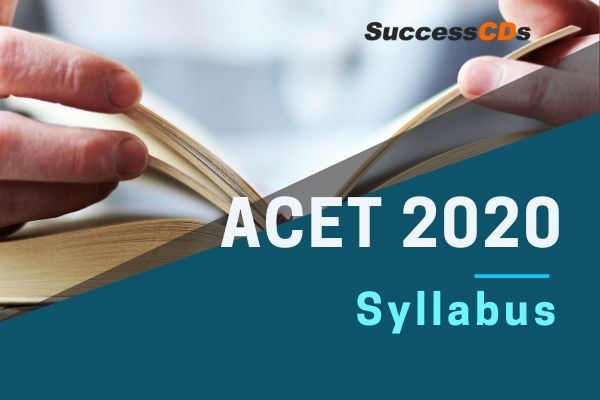# ACET 2020 Syllabus - Actuarial Common Entrance Test## Syllabus for Actuarial Common Entrance Test –ACET 2020

ACET 2020 Syllabus - Institute of Actuaries of India invites application for started the Registration for Actuarial Common Entrance Test (ACET) for February 2020 session. Interested candidates can apply for ACET 2020 through online registrations Process which has begun from 5th December 2019 and will continue till the 28th January 2020.

To qualify as an Actuary, a candidate has to pass all examinations in the prescribed subjects. In addition, he has to comply with other criteria such as experience requirement and attendance at a professionalism course prescribed for the purpose.

Also See : Common Entrance Test

Syllabus for ACET 2020 Examination will include the following

• Mathematics
• Statistics
• English
• Data Interpretation
• Logic

Mathematics

• Notation and standard functions
• Numerical Methods
• Algebra
• Differentiation
• Integration
• Vectors
• Matrices

Statistics

• Permutations and combinations
• Types of data - Statistical diagrams, bar chart, histogram, dot plot, stem-and-leaf, box plot
• Measures of location - mean, median, mode
• Measures of spread - range, interquartile range, standard deviation, variance, Skewness
• Probabilities - basic rules of probabilities
• Advanced probabilities - tree diagrams, conditional probabilities
• Discrete random variables - definitions, probabilities, mean, mode, median, standard deviation, variance, coefficient of skewness
• Continuous random variables - definitions, probabilities, mean, mode, median, standard deviation, variance, coefficient of skewness
• Discrete distributions - discrete uniform, Bernoulli, binomial, Poisson
• Continuous distributions - continuous uniform, exponential, the normal distribution
• Correlation - scatter plots, covariance, correlation coefficient
• Regression

English

• Vocabulary Based (Synonyms Antonyms)
• English Usage or Grammar
• Sentence Correction
• Fill in the blanks
• Cloze Passage
• Analogies or Reverse Analogies
• Jumbled Paragraph
• Meaning-Usage Match
• Summary Questions
• Verbal Reasoning
• Facts / Inferences / Judgements

Vocabulary: Vocabulary questions test the candidate’s knowledge of the primary meanings of words, secondary shades of meaning, usage, idioms and phrases, antonyms, related words, etc.

Grammar: Grammar-based questions test the candidate’s ability to spot and correct grammatical errors. It should generally tests knowledge of high school level grammar and includes areas like subject-verb agreement, use of modifiers, parallel construction, redundancy, phrasal verbs, use of articles, prepositions, etc.

Verbal Reasoning: Verbal reasoning questions are designed to test the candidate’s ability to identify relationships or patterns within groups of words or sentences.

Data Interpretation

Data is given in form of tables, charts and graphs. In this section it is tested that how can you interpret the given data and answers the questions based on it.

• Tables
• Column Graphs
• Bar Graphs
• Line Charts
• Pie Chart
• Venn Diagrams
• Caselet

Combination of two or more types linked to each other.

Logical Reasoning

• Number and Letter Series
• Calendars
• Clocks
• Cubes
• Venn Diagrams
• Binary Logic
• Seating Arrangement
• Logical Sequence
• Logical Matching
• Logical Connectives
• Syllogism
• Blood Relations

### The Structure of ACET Examinations

• ACET is a 3 hour, 100 marks online exam which has 70 multiple choice questions with one correct answer for each question
• The distribution of questions will be:
• 45 questions – 1 mark each
• 20 questions – 2 marks each
• 05 questions – 3 marks each
• After successful registration, students will be able to download the sample question paper for the examination. However, the names of the reference books are available under ACET syllabus tab for the students.
• The Allocation of Marks will be as under:
• Mathematics - 30 marks
• Statistics - 30 marks
• Data Interpretation - 15 marks
• English - 15 marks
• Logical Reasoning - 10 marks
• ACET will be conducted in 24 exam centres in India.
• ACET will be conducted in a single day with one slot.
• There will not be any negative marking for incorrect answers.
• The Pass mark for ACET Examination is 50%.